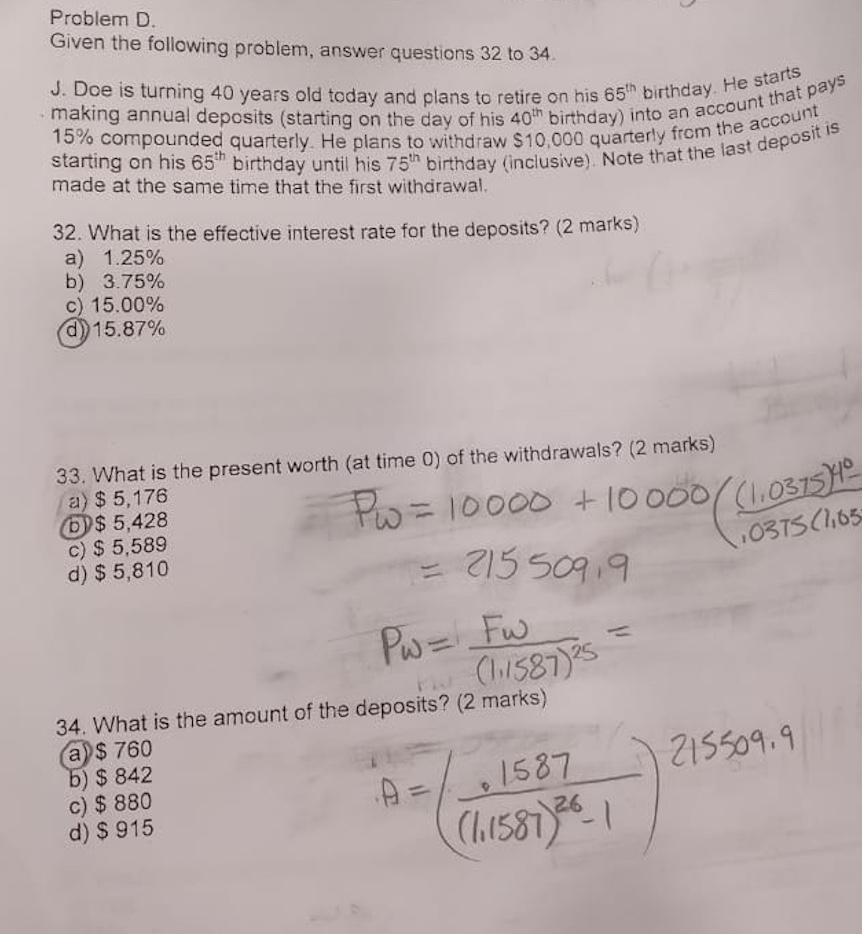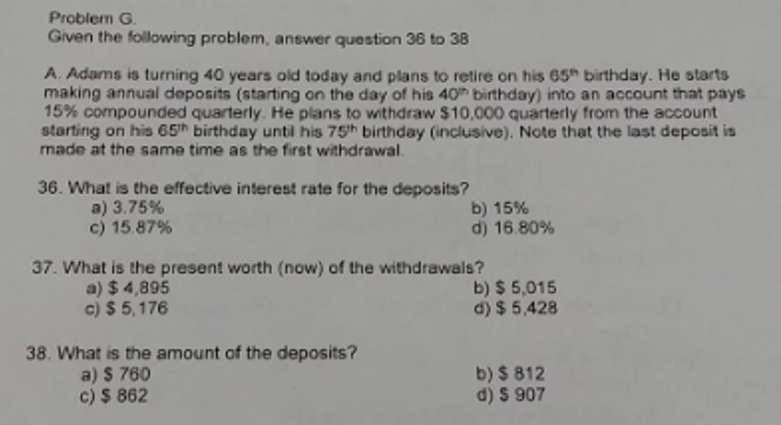# Problem D. Given the following problem, answer questions 32 to 34. J. Doe is turning 40...Problem D. Given the following problem, answer questions 32 to 34. J. Doe is turning 40 years old today and plans to retire on his 65h birthday. He starts 15% compounded quarterly. He plans to withdraw \$10,000 quarterly from the account making annual deposits (starting on the day of his 40th birthday) into an account that pays starting on his 65th birthday until his 75h birthday (inclusive). Note that the last deposit is made at the same time that the first withdrawal. 32. What is the effective interest rate for the deposits? (2 marks) a) 1.25% b) 3.75% c) 15.00% d)15.87% 33. What is the present worth (at time 0) of the withdrawals? (2 marks) a) \$ 5,176 O\$ 5,428 c) \$ 5,589 d) \$ 5,810 Po= 10000 + 10 000(1.0315 15 509 9 0375C65 Pw=Fus C581s 1s 34. What is the amount of the deposits? (2 marks) a)\$ 760 b) \$ 842 c) \$ 880 d) \$ 915 215509.9 A-1587 26 (h1581

(a)

Interest rate is 15% comoounded quarterly.

So effective Interest rate is per quarter is 0.15/4 = 0.0375

Deposit is made Yearly. so effective interest rate per annum formula

Effective interest rate =((1+ quarterly rate)^no of quarter)

((1+ 0.0375)^4)-1 = 0.158650415

So, annual interest rate= 0.1587 or 15.87%

(b)
Quarterly Annuity required from 65th birthday to 75 th birthday. =\$ 10000
75th birthday is included and first withdrawl is made at time of last deposit. So it is Annuity due from 65th to 75th birthday. Number of quarters shall be (1+(10*4))= 41 quarters (as at 65th birthday Amount is withdrawn)
Quarterly Interest rate =   0.0375
We need to Calculate present Value of annuity to Calculate Amount that must have at the time of 17th birthday for Annuity withdrawl of \$4000
Present Value of annuity due formula = Annuity *(1+i)*(1-(1/(1+i)^n))/i
10000*(1+0.0375)*(1-(1/((1+0.0375)^41)))/0.0375
215,509.90
We must have accumulated \$215509.90 at the time of 65th birthday.
To Calculate present worth at (t=0) no. of periods = (65-40)=   25 years.
Future value is \$215509.90 in respect to today time.

Present Value = Future value/(1+i)^n
215509.90/(1+0.158650415)^25

=\$ 5428.210582
So, present worth is \$5428.

(c)
To Calculate annual deposit for future value of \$215509.90, Future value of Annuity formula will become applicable.
Deposit is made from 40th birthday itself to 65th birthday. So Total periods (n) =   26

Future value of annuity formula = P *{ (1+r)^n - 1 } / r
215509.90 = P*(((1+0.158650415)^26)-1)/0.158650415
215509.90/   283.6459214   =P
P =   759.7849419

So, We need to contribute \$760 annually.Problem G Given the following problem, answer question 36 to 38 A. Adams is turning 40 years old today and plans to retire on his 65 birthday. He starts making annual doposits (starting on the day of his 40h birthday) into an account that pays 15% compounded quarterly. He plans to withdraw \$10,000 quarterly from the account starting on his 65h birthday until his 75th birthday (inclusive). Note that the last deposit is made at the same time as the...